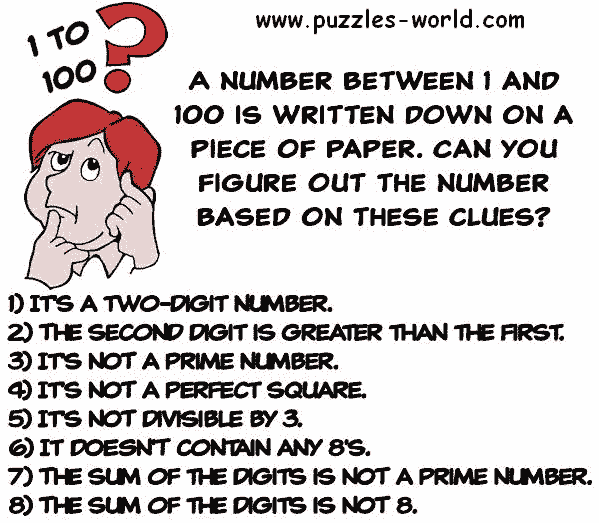# Figure out the number based on the clues## Figure out the number based on the cluesA number between 1 and 100 is written down on a piece of paper.
Can you figure out the number based on these clues ?

1) Its a two-digit number.
2) The second digit is greater than the first.
3) Its not a Prime number.
4) Its not a perfect square.
5) Its not divisible by 3.
6) It doesn't contain any 8's.
7) The sum of the digits is not a prime number.
8) The sum of the digits is not 8.